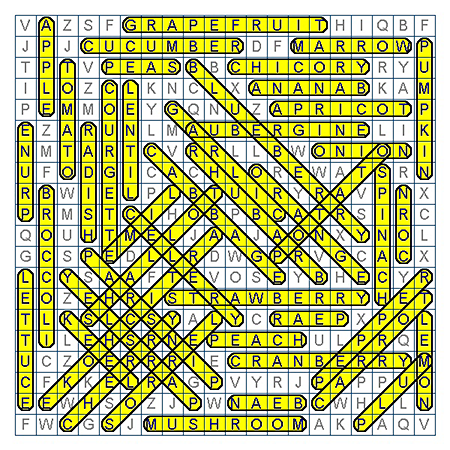## C.2 Project ideas

A successful data science project involves asking a good question, creating or finding data that allow answering it, and possessing the skills and tools for actually doing so. In addition, communicating all this to others requires documenting the process in a transparent fashion.

In the following, we collect some ideas for potential data science projects.

### C.2.1 Conceptual projects

The following projects do not rely on data, but address conceptual problems that combine multiple types of representations:

#### Non-decimal arithmetic

• Create functions that transform integers from our standard decimal notation into symbol strings (i.e., sequences of numerals/digits) that use a positional digit system of a different base (e.g., base 2), and back (from some non-decimal base into base 10). Here are some examples that demonstrate and verify the success of this translation process:
Table C.1: Translating number representations from decimal notation into a different base, and back.
n_org base n_base n_dec same
8242 2 10000000110010 8242 TRUE
719 9 878 719 TRUE
8921 8 21331 8921 TRUE
4921 7 20230 4921 TRUE
604 2 1001011100 604 TRUE
2263 5 33023 2263 TRUE
9985 9 14624 9985 TRUE
74 4 1022 74 TRUE
9253 10 9253 9253 TRUE
9053 5 242203 9053 TRUE
• Add algorithms for arithmetic operations (e.g., for addition, subtraction, multiplication, etc.) that work for numbers written in arbitrary base notations (e.g., with base values of $$2 \leq b \leq 16$$).

• How do particular base values affect the trade-offs (e.g., the frequency of symbols vs. recalling numeric facts from memory) in your calculations? (Comparing the base values of $$10$$, $$11$$, and $$12$$, would yield interesing insights.)

• Add translation functions and arithmetic operations for a non-positional number system. For instance, see the as.roman() function of the utils package (and Schlimm & Neth, 2008).

#### Letter arithmetic

• Use your knowledge on replacing text symbols (see Chapter 9) to create letter arithmetic problems, like the following (from Simon & Newell, 1971):
  DONALD
+ GERALD
--------
ROBERT

Information given: D$$= 5$$.

• We can easily turn arithmetic expressions into letter-arithmetic expressions by simply replacing our common numeral symbols (i.e., the Hindu-Arabic digits $$0-9$$) by alphabetic characters (e.g., using the transl33t() function of ds4psy, see Section 9.5.1). As this neither changes the notational properties of the number system nor the rules of calculation, the difficulty of such problems shows how much we usually rely on our familiarity with particular numerals:
#>  "BIDI + IGAE = GFJFA"
#>  "BIDI - IGAE = -FFHG"
#>  "BIDI * IGAE = FEAFFDIH"
#>  "BIDI / IGAE = J.DEFECJGBGIHCCHE"
Table C.2: Some problems in letter arithmetic.
p_1 p_2 p_3 p_4
4858 BIDI BIDI BIDI BIDI
+ + - * /
8179 IGAE IGAE IGAE IGAE
= = = = =
13037 GFJFA -FFHG FEAFFDIH J.DEFECJGBGIHCCHE
• If we wanted to move further from letter arithmetic to cryptoarithmetic, we can increase the obscurity by translating our numeric representation from a decimal notation to some non-decimal base value (see the project on non-decimal arithmetic above). Interestingly, by reducing the number of symbols involved (for base values $$b < 10$$) and increasing the number of constraints on the calculations shown, this could render the problems easier, rather than more difficult.

• Create an algorithm that can solve cryptoarithmetic problems — and determines whether they have a unique solution.

#### Word search puzzles

• Combine your knowledge on character vectors (e.g., countries or fruits in ds4psy ) and plotting text (e.g., see the plot_chars() and plot_text() functions of ds4psy from Section 9.5.5) to create word search puzzles, like the following :Figure C.1: A word search puzzle (from Payne et al., 2007, doi 10.1037/0096-3445.136.3.370).

Figure C.1 hides the names of $$N = 45$$ fruits or vegetables in a 20 x 20 grid of letters. Note that the target words can be written in various directions.

• Allow for precise specifications of puzzle difficulty (e.g., by providing arguments for the frequency of words, their length, position and direction, as well as the word or letter frequency of targets and distractors).

• How can we guarantee that the distractors do not contain words? Create an algorithm that searches such puzzles for a given dictionary of words.

### C.2.2 Data-based projects

As data-based projects primarily require a suitable dataset, they cannot be discussed independently of data. When starting with a question, it is often necessary to combine multiple datasets to address or answer it.

### References

Payne, S. J., Duggan, G. B., & Neth, H. (2007). Discretionary task interleaving: Heuristics for time allocation in cognitive foraging. Journal of Experimental Psychology: General, 136(3), 370–380. https://doi.org/10.1037/0096-3445.136.3.370
Schlimm, D., & Neth, H. (2008). Modeling ancient and modern arithmetic practices: Addition and multiplication with Arabic and Roman numerals. In B. Love, K. McRae, & V. Sloutsky (Eds.), Proceedings of the 30th Annual Meeting of the Cognitive Science Society (pp. 2097–2102). Retrieved from http://nbn-resolving.de/urn:nbn:de:bsz:352-283870
Simon, H. A., & Newell, A. (1971). Human problem solving: The state of the theory in 1970. American Psychologist, 26(2), 145–159. https://doi.org/10.1037/h0030806